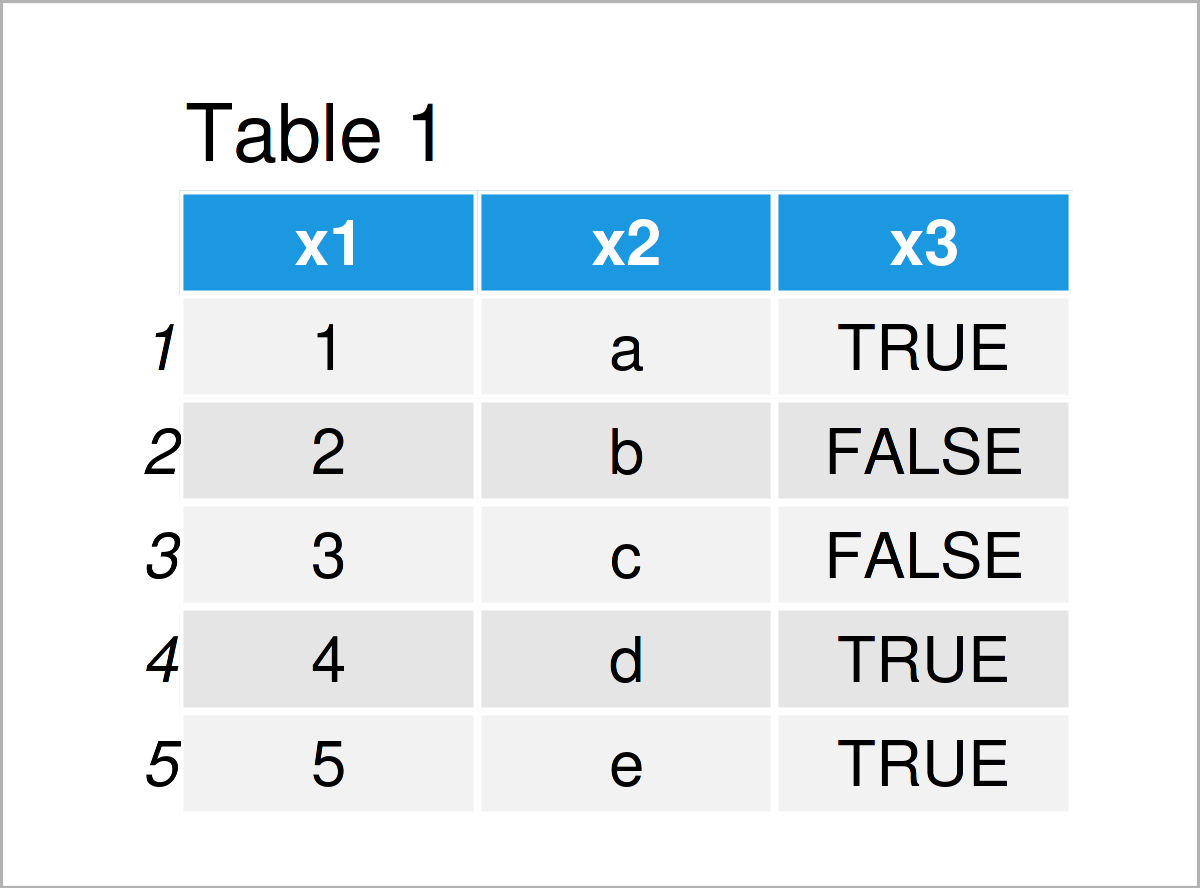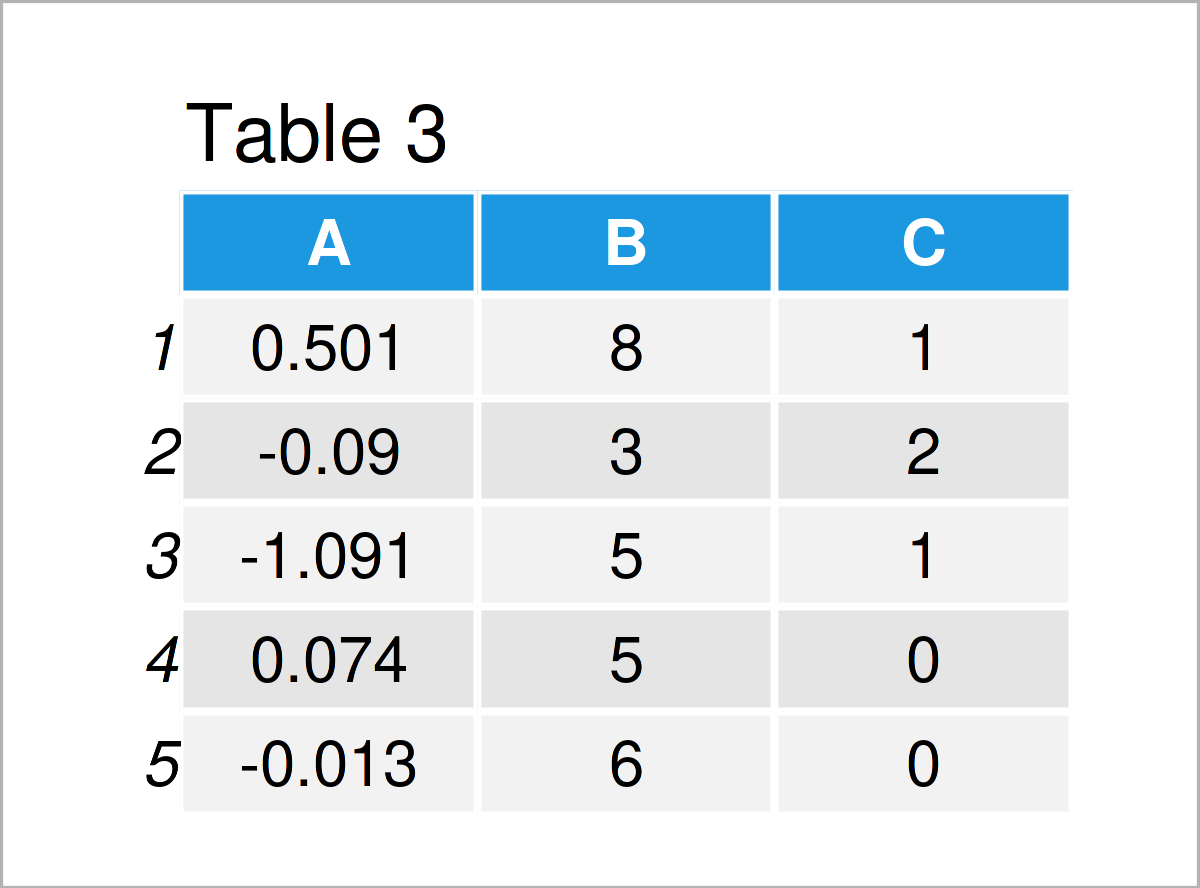# Create data.table in R (3 Examples)

In this R tutorial you’ll learn how to build a data.table object. We also show you how to create a data.table with column names.

The content of the tutorial looks as follows:

Let’s dig in…

## Example Data & Add-On Packages

In order to use the functions of the data.table package, we first need to install and load data.table to RStudio:

```install.packages("data.table")                    # Install data.table package

We also create some example vectors which we later combine to a data.table:

```x1 <- 1:5                                         # Vector 1
x2 <- letters[1:5]                                # Vector 2
x3 <- c(TRUE, FALSE, FALSE, TRUE, TRUE)           # Vector 3```

## Example 1: data.table From Vectors

The following R code shows how to combine the previously defined vectors into a data.table.

```dt_1 <- data.table(x1, x2, x3)                    # Create data.table
dt_1```Have a look at the previous Table 1. The data we created has five rows and three variables, which correspond to our input vectors. The column names correspond to our vector names.

## Example 2: data.table From Vectors With Specific Column Names

In Example 1, we created a data.table where the column names were automatically set to the input vector names. In Example 2, we generate the same data.table, but explicitly determine the names of the columns.

```dt_2 <- data.table("A" = x1, "B" = x2, "C" = x3)  # Create data.table
dt_2```The output of the previous R code is shown in Table 2: Now the columns are called A, B, and C.

## Example 3: data.table With Random Values

Instead of creating a data.table from already existing vectors, we can also make it from scratch. Example 3 explains how to, using randomly generated values from the normal, the poisson, and the binomial distribution.

```dt_3 <- data.table("A" = rnorm(5),
"B" = rpois(5, 5),
"C" = rbinom(5, 2, .6))        # Create data.table
dt_3```As shown in Table 3, we have created a data.table with random values.

## Video, Further Resources & Summary

Do you want to learn more about the generation of a data.table? Then you may have a look at the following video on my YouTube channel. In the video, I’m explaining the R syntax of this post.

Furthermore, you might want to have a look at some other RStudio tutorials on this website. A selection of tutorials that are related to the generation of a data.table is listed below.

In this tutorial you have learned how to you can initialize and construct a data.table object in the R programming language. Please let me know in the comments section below, in case you have additional comments and/or questions.

This page was created in collaboration with Anna-Lena Wölwer. Have a look at Anna-Lena’s author page to get further details about her academic background and the other articles she has written for Statistics Globe.

Subscribe to the Statistics Globe Newsletter